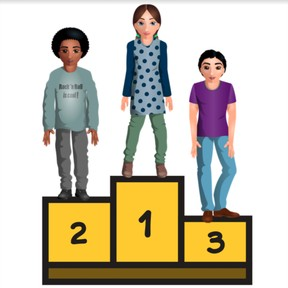Know ordinal numbers to 10

# Know ordinal numbers to 10

Know ordinal numbers to 10

No account needed.8,000 schools use Gynzy92,000 teachers use Gynzy1,600,000 students use Gynzy

## General

Students learn what ordinal numbers are and learn the ordinal numbers from 1 to 10.

K.CC.B.4

## Relevance

Discuss why it is important to learn the ordinal numbers and know them. With ordinal numbers you can set things in order. You can say who ended first, second and third in a race.

## Introduction

Show the number line on the interactive whiteboard. The number line is incomplete, drag the numbers to the right place on the line.

## Development

Explain that ordinal numbers show the order of something. The interactive whiteboard has an image of a row of houses with ordinal numbers below them. Count the houses and name the ordinal number that belongs with them. Say the ordinal numbers and have students pay particular attention to the ordinal numbers which are different than the counting numbers. First, for example, does not sound the same as one, nor does second contain "two". Show the ordinal numbers and count objects one by on, until you reach the underlined object. NExt indicate which ordinal number it gets.

Check that students understand ordinal numbers to 10 by asking the following questions:
When do you use ordinal numbers?
Can you say the ordinal numbers?

## Guided Practice

Students first practice by indicating which ordinal number belongs to the underlined object. Next students are given an image, and have to say what/who is shown by a given ordinal number. Finally they see multiple rows and students have to indicate which row contains the ordinal number they are given.

## Closing

Discuss with students that it is useful to know ordinal numbers. Check that students know that they can use the number line to help determine which ordinal number belongs where. To check that students have understood, they have to indicate which vehicle is the fifth. They also have an exercise where a few students are called to the front and the rest of the class must indicate who is second, and who is fourth.

## Teaching Tip

Students who have difficulty with the ordinal numbers can also practice counting on the number line to 10.

### The online teaching platform for interactive whiteboards and displays in schools

• Save time building lessons

• Manage the classroom more efficiently

• Increase student engagement